# MAXA Function

Returns the largest value in a given list of arguments

## What is the MAXA Function?

The MAXA Function is categorized under Excel Statistical functions. MAXA will return the largest value in a given list of arguments. From a given set of numeric values, it will return the largest value. The function compares numbers, text, and logical values as TRUE or FALSE.

In financial modeling, the MAXA function can be useful in extracting the maximum numeric value, ignoring empty cells. For example, we can calculate the highest test score, or the fastest runner time in a race, the highest temperature, or the largest expense or sales amount, etc.

### Formula

=MAXA(value1, [value2], …)

Value1 and value2 are the arguments used for the MAXA function, where Value1 is required and the subsequent values are optional.

In MS Excel 2007 and later versions, we can provide up to 255 number arguments to the function. However, in Excel 2003 and earlier versions, it can only accept up to 30 number arguments.

Arguments can be provided as constants, or as cell references or ranges. Also, the MAXA function evaluates TRUE and FALSE values as 1 and 0, respectively.

### How to use the MAXA Function in Excel?

As a worksheet function, the MAXA function can be entered as part of a formula in a cell of a worksheet. To understand the uses of the function, let us consider a few examples:

#### Example 1

Let us calculate the highest market expense in the given zones: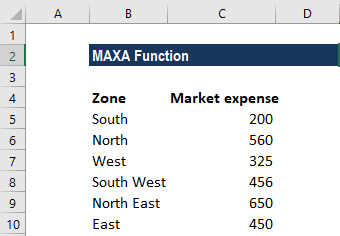The formula used is below: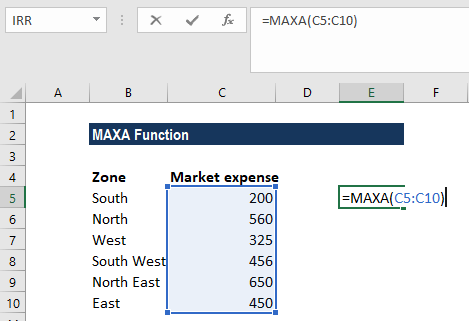We get 650 as the result, which the largest expense in the given range.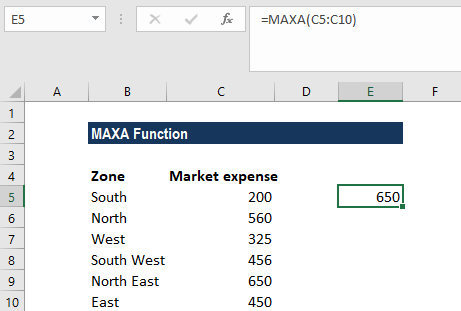#### Example 2

Suppose we are given the following data: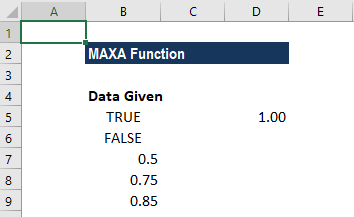Using the function, we can retrieve the largest value from the set of values in cells A2-A6.

When we give the formula: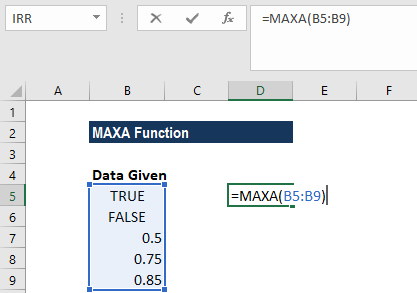We get the result below: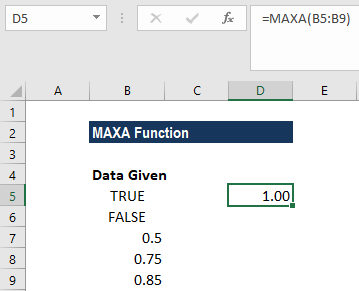We get 1 as the result. The MAXA function treated the value TRUE in cell A2 as the numeric value 1. Therefore, it is the highest value in the range A1-A6.

### A few things to remember about the MAXA Function

1. #VALUE! error – Occurs if any values that are supplied directly to the MAXA function are non-numeric.
2. The MAXA function evaluates TRUE and FALSE values as 1 and 0, respectively.
3. The primary difference between MAX and MAXA is that the “A” version evaluates TRUE and FALSE values as 1 and 0, respectively.

Thanks for reading CFI’s guide to important Excel functions! By taking the time to learn and master these functions, you’ll significantly speed up your financial analysis. To learn more, check out these additional CFI resources:

• Excel Functions for Finance